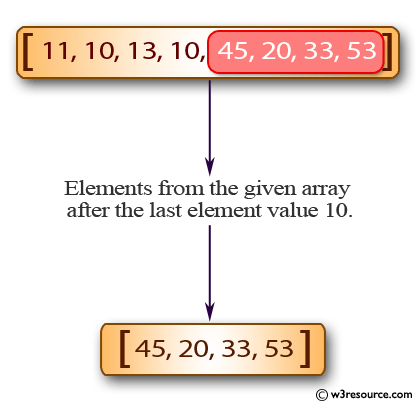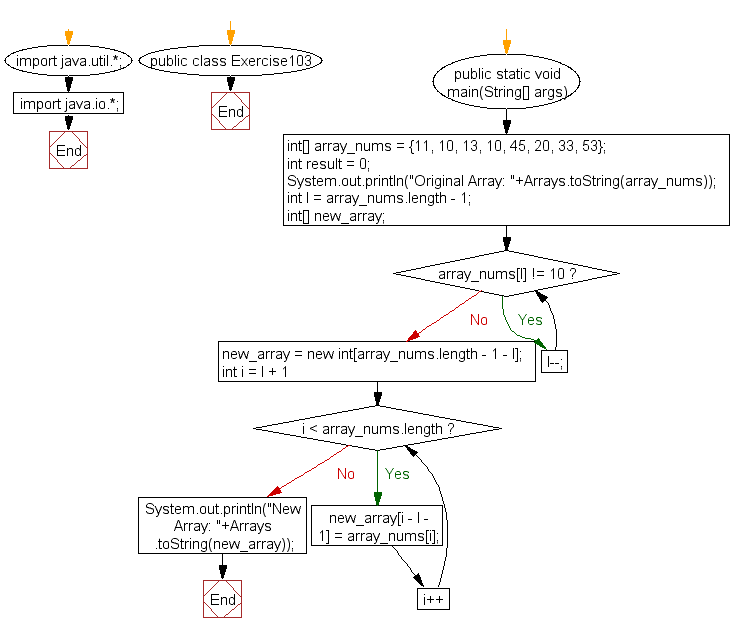﻿ Java: Make an array with the elements from an array after 10# Java Exercises: Create a new array from a given array of integers, new array will contain the elements from the given array after the last element value 10

## Java Basic: Exercise-103 with Solution

Write a Java program to create a new array from a given array of integers, new array will contain the elements from the given array after the last element value 10.

Pictorial Presentation:Sample Solution:

Java Code:

``````import java.util.*;
import java.io.*;
public class Exercise103 {
public static void main(String[] args)
{
int[] array_nums = {11, 10, 13, 10, 45, 20, 33, 53};
int result = 0;
System.out.println("Original Array: "+Arrays.toString(array_nums));

int l = array_nums.length - 1;
int[] new_array;
while(array_nums[l] != 10)
l--;
new_array = new int[array_nums.length - 1 - l];
for(int i = l + 1; i < array_nums.length; i++)
new_array[i - l - 1] = array_nums[i];
System.out.println("New Array: "+Arrays.toString(new_array));
}
}
```
```

Sample Output:

```Original Array: [11, 10, 13, 10, 45, 20, 33, 53]
New Array: [45, 20, 33, 53]
```

Flowchart:Java Code Editor:

What is the difficulty level of this exercise?

Test your Programming skills with w3resource's quiz.

﻿

## Java: Tips of the Day

countOccurrences

Counts the occurrences of a value in an array.

Use Arrays.stream().filter().count() to count total number of values that equals the specified value.

```public static long countOccurrences(int[] numbers, int value) {
return Arrays.stream(numbers)
.filter(number -> number == value)
.count();
}
```

Ref: https://bit.ly/3kCAgLb